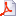# Vanessa Mattesi defended her PhD

Wave propagation in a domain including small heterogeneities: asymptotic modeling and nmerics

In this thesis, we focus our attention on the modeling of heterogeneities which are smaller than the wavelength. The document is decomposed into two parts : a theoretical one and a numerical one. In the first part, we derive a matched asymptotic expansion composed of a far-field expansion and a near-field expansion. The terms of the far-field expansion are singular solutions of the wave equation whereas the terms of the near-field expansion satisfy quasistatic problems. These expansions are matched in an intermediate region. We justify mathematically this theory by proving error estimates.
In the second part, we describe the Discontinuous Galerkin method, a local time stepping method and the implementation of the matched asymptotic method. Numerical simulations illustrate these results.Publications HAL de mattesi du labo/EPI magique-3d

titre
Propagation des ondes dans un domaine comportant des petites hétérogénéités : modélisation asymptotique et calcul numérique
auteur
Vanessa Mattesi
article
Analyse numérique [math.NA]. Université de Pau et des pays de l’Adour, 2014. Français
Accès au texte intégral et bibtex